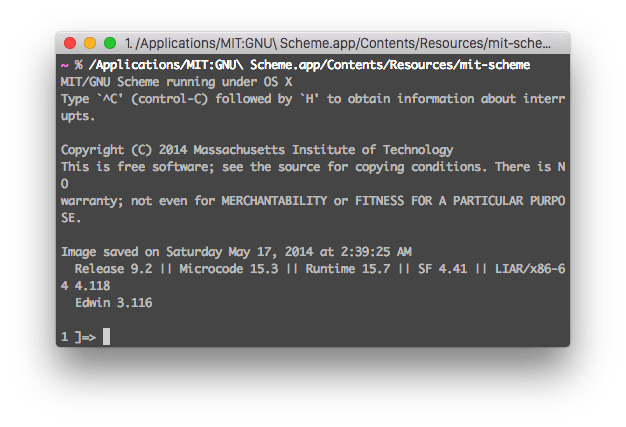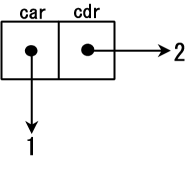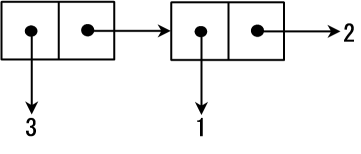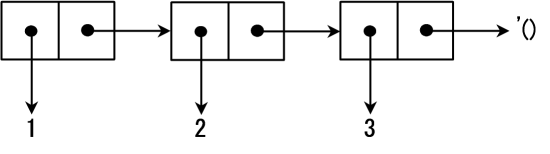# OneV's Den

## 成为 Scheme Hacker 的第一步## Hello 1 + 1

``````1 ]=> (+ 1 1)

;Value: 2

1 ]=>
``````

`1 ]=> ` 是输入提示符，我们输入的内容是 `(+ 1 1)`，得到的结果是 2。虽然语句很简单，但是这里包含了 Scheme 的最基本的语素，有三个地方值得特别注意。

1. 成对的括号。一对括号表示的是一步计算，这里 `(+ 1 1)` 表示的就是 1 + 1 这一步运算。
2. 紧接括号的是函数名字，再然后是函数的参数。在这里，函数名字就是 “+”，两个 1 是它的参数。Scheme 中大部分的运算符其实都是函数。
3. 使用空格，tab 或是换行符来分割函数名以及参数。

``````1 ]=> (* 2 3)

;Value: 6

1 ]=>
``````

``````1 ]=> (- 10 5 3)

;Value: 2

1 ]=> (/ 20 2 2)

;Value: 5
``````

``````1 ]=> (/ 10 3)

;Value: 10/3
``````

``````1 ]=> (/ 2)

;Value: 1/2
``````

``````1 ]=> (exact->inexact (/ 10 3))

;Value: 3.3333333333333335
``````

Scheme 也内建定义了一些其他的数学运算符号，如果你感兴趣，可以查看 R6RS 的相关章节

R6RS (Revisedn Report on the Algorithmic Language Scheme, Version 6) 是当前的 Scheme 标准。

## 定义变量和方法，Hello World

Scheme 中通过 `define` 来定义变量和方法：

``````; s 是一个变量，值为 "Hello World"
(define s "Hello World")

; f 是一个函数，它不接受参数，调用时返回 "Hello World"
(define f (lambda () "Hello World"))
``````

``````1 ]=> (define s "Hello World")

;Value: s

1 ]=> (define f (lambda () "Hello World"))

;Value: f

1 ]=> s

;Value 24: "Hello World"

1 ]=> f

;Value 25: #[compound-procedure 25 f]

1 ]=> (f)

;Value 26: "Hello World"
``````

``````1 ]=> (define hello
(lambda (name)
(string-append "Hello " name "!")
)
)

;Value: hello

1 ]=> (hello "onevcat")

;Value 27: "Hello onevcat!"
``````

``````1 ]=> (define (hello name)
(string-append "Hello " name "!"))

;Value: hello

1 ]=> (hello "onevcat")

;Value 28: "Hello onevcat!"
``````

## 条件分支和布尔逻辑

``````(if condition ture_action false_action)
``````

``````1 ]=> (define (abs input)
(if (< input 0) (- input) input))

;Value: abs

1 ]=> (abs 100)

;Value: 100

1 ]=> (abs -100)

;Value: 100
``````

``````1 ]=> (and #f 0)

;Value: #f

1 ]=> (and 1 2 "Hello")

;Value 13: "Hello"

1 ]=> (or #f 0)

;Value: 0

1 ]=> (or 1 2 "Hello")

;Value: 1

1 ]=> (or #f #f #f)

;Value: #f
``````

``````(define (pro3and a b c)
(and (positive? a)
(positive? b)
(positive? c)
(* a b c)
)
)
``````

``````(cond
(predicate_1 clauses_1)
(predicate_2 clauses_2)
......
(predicate_n clauses_n)
(else        clauses_else))
``````

`begin` 将顺次执行一系列语句：

``````(define (foo)
(begin
(display "hello")
(newline)
(display "world")
)
)
``````

`when` 当条件满足时执行一系列代码，而 `unless` 在条件不满足时执行一系列代码。这些改动可以看出一些现代脚本语言的特色，但是新的标准据说也在 Scheme 社区造成了不小争论。虽然结合使用 `if``and``or` 肯定是可以写出等效的代码的，但是这些额外的分支控制语句确实增加了语言的便利性。

## 循环

### do

``````1 ]=> (do ((i 0 (+ i 1))) ; 初始值和 step 条件
((> i 4))       ; 停止条件，取值为 #f 时停止
(display i)       ; 循环主体 (命令)
)
01234
;Value: #t
``````

### 递归

``````1 ]=> (define (count n)
(and (display (- 4 n))
(if (= n 0) #t (count (- n 1)))
)
)

;Value: count

1 ]=> (count 4)
01234
;Value: #t
``````

### 列表和递归``````1 ]=> (cons 1 2)

;Value 13: (1 . 2)
``````

``````1 ]=> (car (cons 1 2))

;Value: 1

1 ]=> (cdr (cons 1 2))

;Value: 2
``````

cons cell 每个节点的内容可以是任意的数据类型。一种最常见的结构是 `car` 中是数据，而 `cdr` 指向另一个 cons cell：``````1 ]=> (cons 3 (cons 1 2))

;Value 14: (3 1 . 2)
````````````1 ]=>  '(1 2 3)

;Value 15: (1 2 3)

1 ]=> (car '(1 2 3))

;Value: 1

1 ]=> (cdr '(1 2 3))

;Value 16: (2 3)
``````

``````(define (ins_ls ls)
(if (null? ls)
'()
(cons (+ (car ls) 1) (ins_ls (cdr ls)))
)
)

(ins_ls '(1 2 3 4 5))

;=> (2 3 4 5 6)
``````

### 尾递归

``````(define (ins_ls ls)
(ins_ls_interal ls '()))

(define (ins_ls_interal ls ls0)
(if (null? ls)
ls0
(ins_ls_interal (cdr ls) (cons ( + (car ls) 1) ls0))))

(define (rev_ls ls)
(rev_ls_internal ls '()))

(define (rev_ls_internal ls ls0)
(if (null? ls)
ls0
(rev_ls_internal (cdr ls) (cons (car ls) ls0))))

(rev_ls (ins_ls '(1 2 3 4 5)))

;=> (2 3 4 5 6)
``````

## 函数式

``````1 ]=> (sort '(7883 9099 6729 2828 7754 4179 5340 2644 2958 2239) <)

;Value 13: (2239 2644 2828 2958 4179 5340 6729 7754 7883 9099)
``````

``````1 ]=> (sort '(7883 9099 6729 2828 7754 4179 5340 2644 2958 2239)
(lambda (x y) (< (modulo x 100) (modulo y 100))))

;Value 14: (2828 6729 2239 5340 2644 7754 2958 4179 7883 9099)
``````

``````(map (lambda (x) (+ x 1)) '(1 2 3 4 5))

;=> (2 3 4 5 6)
``````